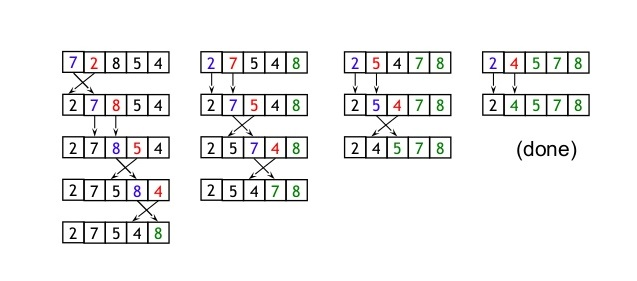# Implementation in C

suggest change

Bubble sort is also known as Sinking Sort. It is a simple sorting algorithm that repeatedly steps through the list to be sorted, compares each pair of adjacent items and swaps them if they are in the wrong order.

Bubble sort exampleImplementation of Bubble Sort I used C# language to implement bubble sort algorithm

``````public class BubbleSort
{
public static void SortBubble(int[] input)
{
for (var i = input.Length - 1; i >= 0; i--)
{
for (var j = input.Length - 1 - 1; j >= 0; j--)
{
if (input[j] <= input[j + 1]) continue;
var temp = input[j + 1];
input[j + 1] = input[j];
input[j] = temp;
}
}
}

public static int[] Main(int[] input)
{
SortBubble(input);
return input;
}
}``````